# KoolReport's Forum

Official Support Area, Q&As, Discussions, Suggestions and Bug reports.

# Cube and chartjs options #1141

pargibay opened this topic on on Oct 31, 2019 - 21 comments

pargibay commented on Oct 31, 2019

Hi, How can i apply column settings in chartjs if i am using cube? I need to use the "spanGaps" property but I don't know how to do it. Thank you Regards

David Winterburn commented on Nov 4, 2019

Hi,

Which column setting did you want to use? For Chartjs, we have the `columns` property to set up columns to draw and their setting. Here's an example:

``````             LineChart::create(array(
"title"=>"Stepped Line Chart",
"dataSource"=>\$time_sale,
"columns"=>array(
"month",
"sale"=>array(
"label"=>"Sale",
"type"=>"number",
"prefix"=>"\$",
"config"=>array(
"steppedLine"=>true,
)
),
),
));
``````

Let us know if you want something else. Thanks!

pargibay commented on Nov 4, 2019

Hello david, Thanks for your answer. I know it this way (it's in the documentation) but I use the cube to get columns with non-fixed db names, so I can't define columns in this way, but I need to define properties for these columns Thanks Regards

pargibay commented on Nov 11, 2019

UP

KoolReport commented on Nov 12, 2019

Hi pargibay, you can get all columns name from dataStore, for example:

``````\$columnNames = array_keys(\$this->dataStore("data-from-cube")->meta()["columns"]);
``````

Now with all the name, you can construct a "columns" array:

``````\$columns = array();
foreach(\$columnNames as \$name)
{
\$columns[\$name] = array(
"label"=>ucfirst(\$name)
);
}
``````

Basically you can base on the name to add customized property for each columns and then later you can put into any widget of KoolReport:

``````LineChart::create(array(
"dataSource"=>\$this->dataStore("data-from-cube"),
"columns"=>\$columns
));
``````

Hope that helps.

pargibay commented on Nov 13, 2019

Hi koolreport

Thank you, it is working but now the "config" => "spanGap" option is not recognized while, for example, "steppedLine" is working.

I print the column array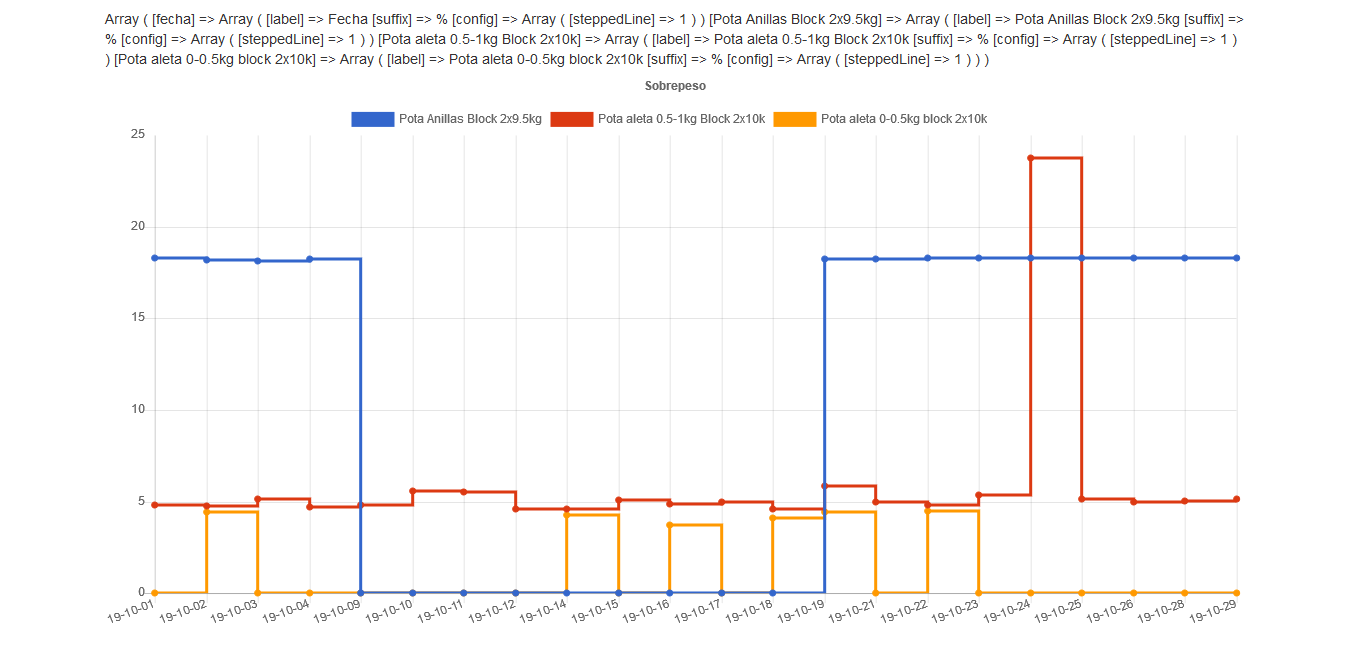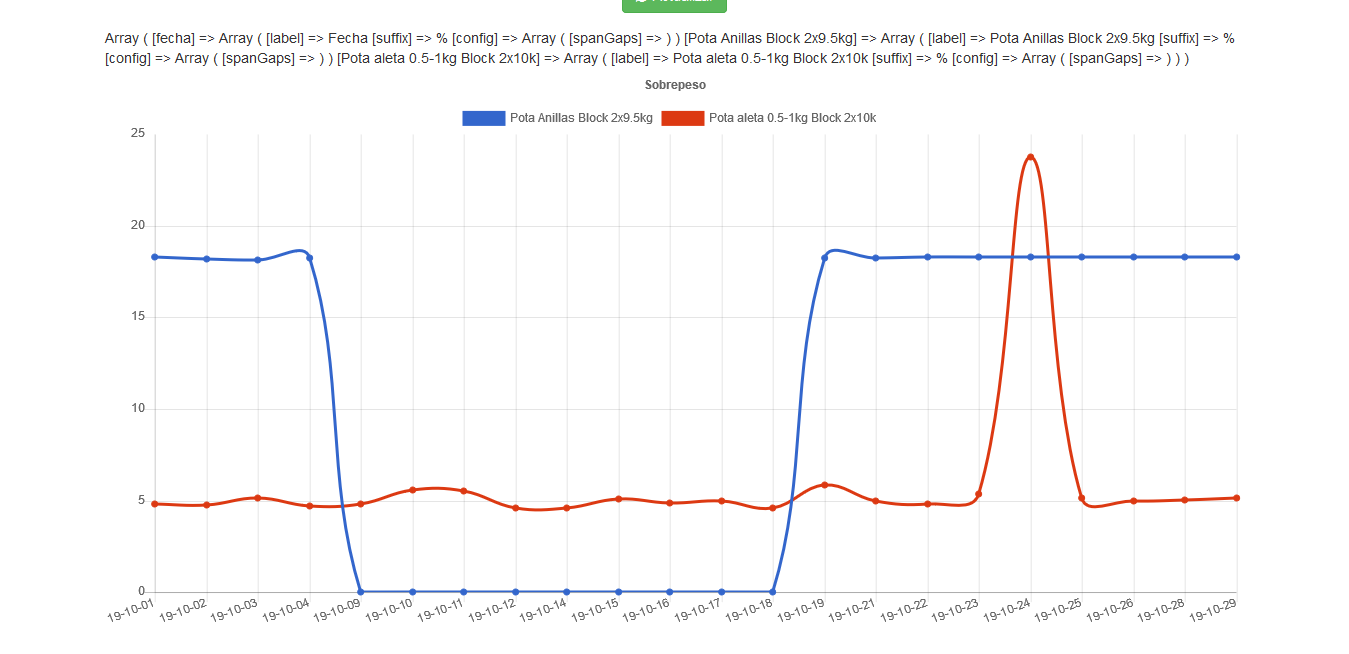KoolReport commented on Nov 13, 2019

pargibay commented on Nov 13, 2019

Hi, Of course:

``````\$this->src("mssql")->query("SELECT m.code AS codigo,FORMAT(pk.prday,'yy-MM-dd') AS fecha,m.name AS producto,ROUND(pk.nominal, 2) AS kg_un,SUM(1) AS cantidad,ROUND(SUM(pk.nominal), 2) AS Kgs, ROUND(100 * AVG((pk.weight - pk.nominal)/ pk.nominal), 2) AS overweight FROM dbo.proc_packs AS pk INNER JOIN dbo.proc_materials AS m ON pk.material = m.material INNER JOIN dbo.proc_lots AS l ON pk.lot = l.lot WHERE (pk.prday BETWEEN CONVERT(datetime,:start,21) AND CONVERT(datetime,:end,21)) AND (pk.rtype <> 4) AND (m.code > '800000') AND m.name IN :products GROUP BY m.code, m.name, pk.prday, pk.nominal ORDER BY pk.prday")
->params(array(
":start"=>\$this->params["dateRange"],
":end"=>\$this->params["dateRange"],
":products"=>\$this->params["products"],
))
->pipe(new Cube(array(
"row"=>"fecha",
"columns"=>"producto",
"sum"=>"overweight",
)))
->pipe(new RemoveColumn(array(
"{{all}}"
)))
->pipe(\$this->dataStore('overweight'));
``````

the view

``````\$columnNames = array_keys(\$this->dataStore("overweight")->meta()["columns"]);
\$columns = array();
foreach(\$columnNames as \$name)
{
\$columns[\$name] = array(
"label"=>ucfirst(\$name),
"suffix"=>"%",
"config"=>array("spanGaps"=>false),
);
}
\$dataStore = \$this->dataStore('overweight');
LineChart::create(array(
"title"=>"Sobrepeso",
"dataSource"=>\$dataStore,
"columns"=>\$columns,
));
``````
KoolReport commented on Nov 13, 2019

You do:

``````foreach(\$columnNames as \$name)
{
if(\$name!="fetcha")
\$columns[\$name] = array("label"=>ucfirst(\$name));
else
\$columns[\$name] = array(
"label"=>ucfirst(\$name),
"suffix"=>"%",
"config"=>array("spanGaps"=>false),
);
}
``````

Let us know if it works.

pargibay commented on Nov 14, 2019

Hi,

It is not working, I got the same result.

maybe it is a bug?

pargibay commented on Nov 19, 2019

UP

KoolReport commented on Nov 19, 2019

Please try again with this code:

``````foreach(\$columnNames as \$name)
{
if(\$name=="fetcha")
\$columns[\$name] = array("label"=>ucfirst(\$name));
else
\$columns[\$name] = array(
"label"=>ucfirst(\$name),
"suffix"=>"%",
"config"=>array("spanGaps"=>false),
);
}
``````

Let me know the result

pargibay commented on Nov 19, 2019

Hi Koolreport,

I had assumed that the conditional was "==" and not "!="

The problem is in spamGaps config because if I use other like steppedLine or showLine it is working

Regards

``````foreach(\$columnNames as \$name)
{
if(\$name=="fetcha") //conditional
\$columns[\$name] = array("label"=>ucfirst(\$name));
else
\$columns[\$name] = array(
"label"=>ucfirst(\$name),
"suffix"=>"%",
"config"=>array("spanGaps"=>false),
);
}
``````
KoolReport commented on Nov 20, 2019

I think it is because your data has value 0 so the chartJs still consider a legitimate value to draw line. Setting spanGaps to `false` work the `null` value only (create the gap). So the solution is that you replace all the 0 value with `null`.

pargibay commented on Nov 21, 2019

Hi Koolreport,

I have tried this: force 0 to null with formatValue:

``````\$columnNames = array_keys(\$this->dataStore("overweight")->meta()["columns"]);
\$columns = array();
foreach(\$columnNames as \$name)
{
if(\$name=="fecha")
\$columns[\$name] = array("label"=>ucfirst(\$name));
else
\$columns[\$name] = array(
"label"=>ucfirst(\$name),
"formatValue"=>function(\$value){if(\$value==0){return NULL;}else{return number_format(\$value,2)."%";}},//NULL if value==0
"config"=>array("spanGaps"=>false),
);
}
``````

And this is the result: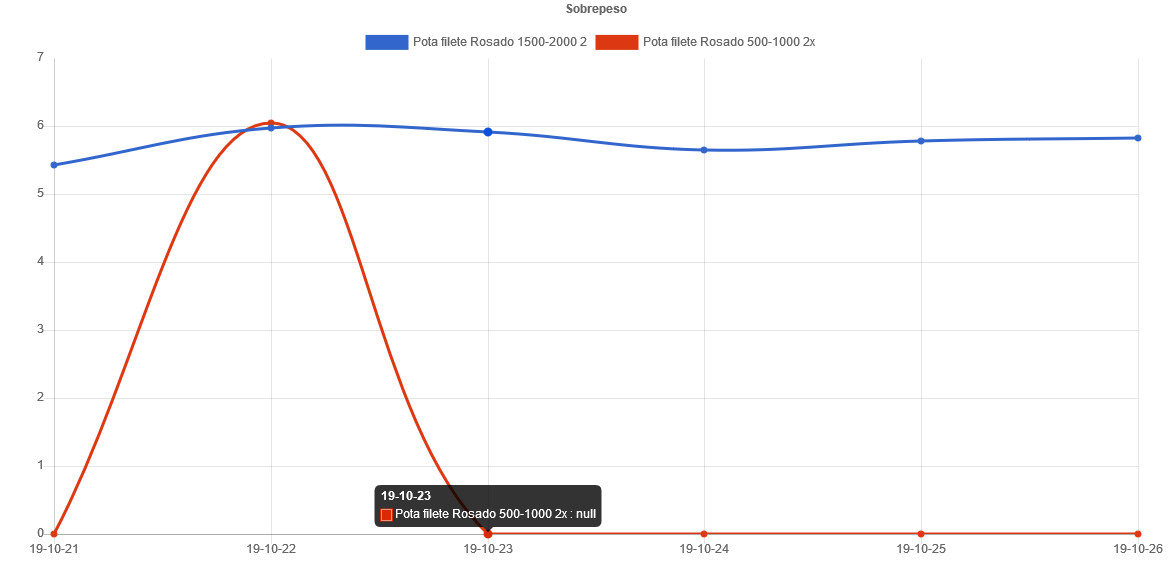KoolReport commented on Nov 21, 2019

You do this in the `setup()` method:

``````...
->pipe(new RemoveColumn(array(
"{{all}}"
)))
->pipe(new \koolreport\cleandata\FillNull(array(
"targetValue"=>0,
"newValue"=>null,
)))
->pipe(\$this->dataStore('overweight'));
``````

Basically the FillNull will target all 0 value and replace with NULL value.

Please try and let us know.

pargibay commented on Nov 21, 2019

Hi, If I set "newValue"=>"NULL" it works (no quotes give error) but there is a warning: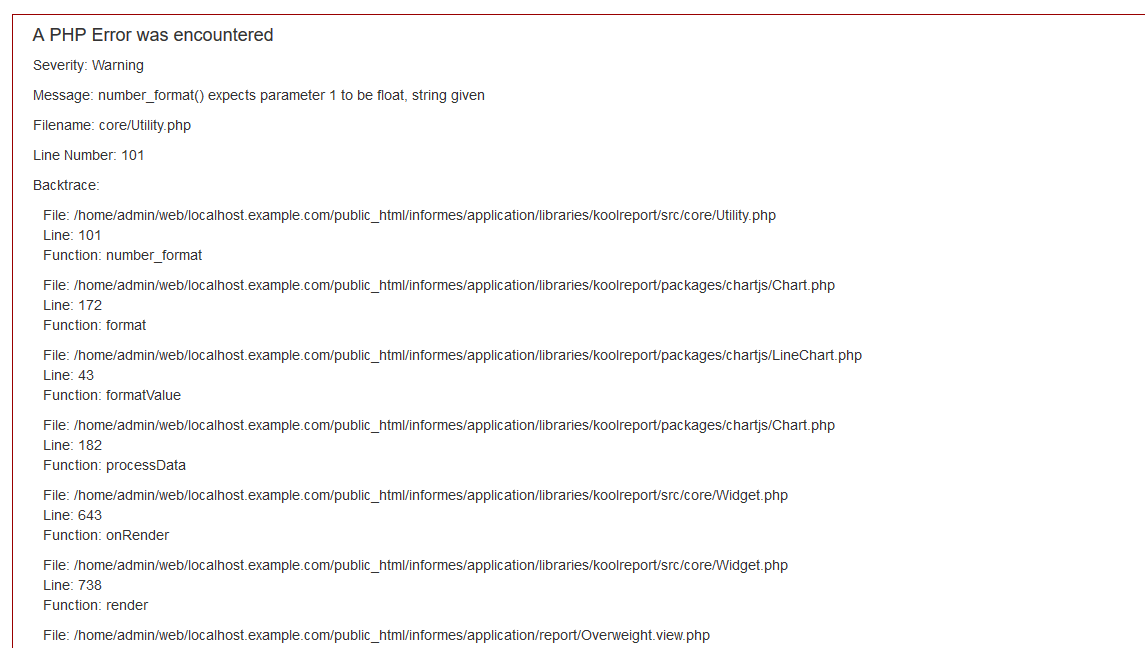KoolReport commented on Nov 21, 2019

You should set the null value, not the string "NULL", please set like this `"newValue"=>null`

pargibay commented on Nov 21, 2019

If I set "newValue"=>null :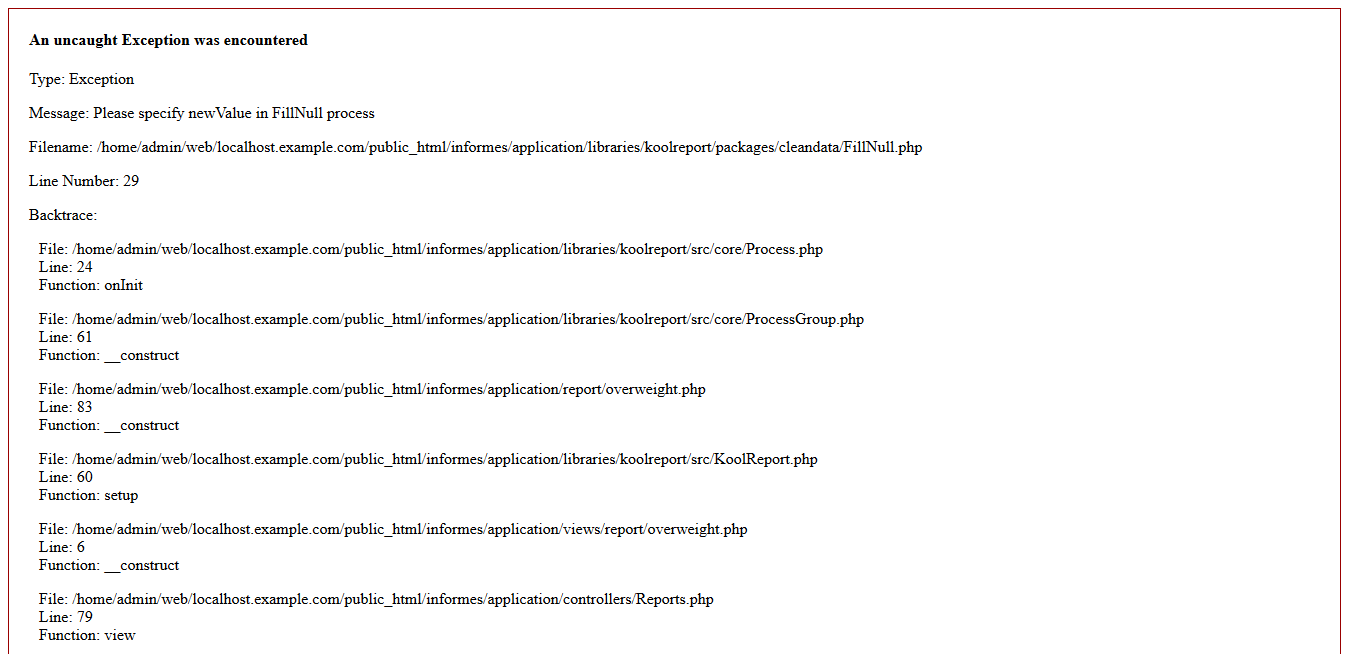KoolReport commented on Nov 21, 2019

Nevermind, you do like following

``````...
->pipe(new RemoveColumn(array(
"{{all}}"
)))
->pipe(new \koolreport\processes\Custom(function(\$row){
foreach(\$row as \$k=>\$v)
if(\$v===0) \$row[\$k]=null;
return \$row;
}))
->pipe(\$this->dataStore('overweight'));
``````

Let me know if it works.

pargibay commented on Nov 21, 2019

It works perfect Thank you very much!

KoolReport commented on Nov 21, 2019